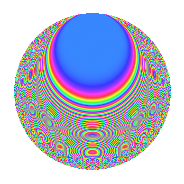Properties

 Label 2672.2.aLevel 2672 Weight 2 Character orbit a Rep. character $$\chi_{2672}(1,\cdot)$$ Character field $$\Q$$ Dimension 83 Newforms 16 Sturm bound 672 Trace bound 7

Related objects

Defining parameters

 Level: $$N$$ = $$2672 = 2^{4} \cdot 167$$ Weight: $$k$$ = $$2$$ Character orbit: $$[\chi]$$ = 2672.a (trivial) Character field: $$\Q$$ Newforms: $$16$$ Sturm bound: $$672$$ Trace bound: $$7$$ Distinguishing $$T_p$$: $$3$$, $$7$$

Dimensions

The following table gives the dimensions of various subspaces of $$M_{2}(\Gamma_0(2672))$$.

Total New Old
Modular forms 342 83 259
Cusp forms 331 83 248
Eisenstein series 11 0 11

The following table gives the dimensions of the cuspidal new subspaces with specified eigenvalues for the Atkin-Lehner operators and the Fricke involution.

$$2$$$$167$$FrickeDim.
$$+$$$$+$$$$+$$$$21$$
$$+$$$$-$$$$-$$$$21$$
$$-$$$$+$$$$-$$$$26$$
$$-$$$$-$$$$+$$$$15$$
Plus space$$+$$$$36$$
Minus space$$-$$$$47$$

Trace form

 $$83q$$ $$\mathstrut +\mathstrut 2q^{3}$$ $$\mathstrut -\mathstrut 2q^{5}$$ $$\mathstrut +\mathstrut 2q^{7}$$ $$\mathstrut +\mathstrut 79q^{9}$$ $$\mathstrut +\mathstrut O(q^{10})$$ $$83q$$ $$\mathstrut +\mathstrut 2q^{3}$$ $$\mathstrut -\mathstrut 2q^{5}$$ $$\mathstrut +\mathstrut 2q^{7}$$ $$\mathstrut +\mathstrut 79q^{9}$$ $$\mathstrut +\mathstrut 2q^{11}$$ $$\mathstrut -\mathstrut 2q^{13}$$ $$\mathstrut +\mathstrut 12q^{15}$$ $$\mathstrut -\mathstrut 2q^{17}$$ $$\mathstrut +\mathstrut 10q^{19}$$ $$\mathstrut +\mathstrut 8q^{23}$$ $$\mathstrut +\mathstrut 77q^{25}$$ $$\mathstrut +\mathstrut 20q^{27}$$ $$\mathstrut +\mathstrut 6q^{29}$$ $$\mathstrut +\mathstrut 10q^{31}$$ $$\mathstrut -\mathstrut 8q^{33}$$ $$\mathstrut -\mathstrut 2q^{37}$$ $$\mathstrut +\mathstrut 4q^{39}$$ $$\mathstrut +\mathstrut 6q^{41}$$ $$\mathstrut +\mathstrut 12q^{43}$$ $$\mathstrut -\mathstrut 10q^{45}$$ $$\mathstrut -\mathstrut 10q^{47}$$ $$\mathstrut +\mathstrut 83q^{49}$$ $$\mathstrut -\mathstrut 20q^{51}$$ $$\mathstrut +\mathstrut 6q^{53}$$ $$\mathstrut +\mathstrut 12q^{55}$$ $$\mathstrut -\mathstrut 8q^{57}$$ $$\mathstrut -\mathstrut 10q^{61}$$ $$\mathstrut -\mathstrut 14q^{63}$$ $$\mathstrut -\mathstrut 4q^{65}$$ $$\mathstrut -\mathstrut 28q^{67}$$ $$\mathstrut -\mathstrut 4q^{71}$$ $$\mathstrut -\mathstrut 2q^{73}$$ $$\mathstrut +\mathstrut 26q^{75}$$ $$\mathstrut +\mathstrut 8q^{77}$$ $$\mathstrut +\mathstrut 8q^{79}$$ $$\mathstrut +\mathstrut 59q^{81}$$ $$\mathstrut -\mathstrut 8q^{83}$$ $$\mathstrut -\mathstrut 28q^{85}$$ $$\mathstrut -\mathstrut 12q^{87}$$ $$\mathstrut -\mathstrut 2q^{89}$$ $$\mathstrut +\mathstrut 20q^{91}$$ $$\mathstrut -\mathstrut 16q^{93}$$ $$\mathstrut -\mathstrut 12q^{95}$$ $$\mathstrut -\mathstrut 2q^{97}$$ $$\mathstrut -\mathstrut 2q^{99}$$ $$\mathstrut +\mathstrut O(q^{100})$$

Decomposition of $$S_{2}^{\mathrm{new}}(\Gamma_0(2672))$$ into irreducible Hecke orbits

Label Dim. $$A$$ Field CM Traces A-L signs $q$-expansion
$$a_2$$ $$a_3$$ $$a_5$$ $$a_7$$ 2 167
2672.2.a.a $$1$$ $$21.336$$ $$\Q$$ None $$0$$ $$0$$ $$3$$ $$-1$$ $$-$$ $$-$$ $$q+3q^{5}-q^{7}-3q^{9}-2q^{13}-2q^{17}+\cdots$$
2672.2.a.b $$2$$ $$21.336$$ $$\Q(\sqrt{5})$$ None $$0$$ $$-3$$ $$0$$ $$7$$ $$+$$ $$-$$ $$q+(-1-\beta )q^{3}+(1-2\beta )q^{5}+(4-\beta )q^{7}+\cdots$$
2672.2.a.c $$2$$ $$21.336$$ $$\Q(\sqrt{13})$$ None $$0$$ $$-1$$ $$-6$$ $$-3$$ $$-$$ $$-$$ $$q-\beta q^{3}-3q^{5}+(-1-\beta )q^{7}+\beta q^{9}+\cdots$$
2672.2.a.d $$2$$ $$21.336$$ $$\Q(\sqrt{2})$$ None $$0$$ $$0$$ $$2$$ $$6$$ $$-$$ $$+$$ $$q+2\beta q^{3}+(1+\beta )q^{5}+3q^{7}+5q^{9}+\cdots$$
2672.2.a.e $$2$$ $$21.336$$ $$\Q(\sqrt{5})$$ None $$0$$ $$1$$ $$-2$$ $$0$$ $$-$$ $$-$$ $$q+\beta q^{3}-q^{5}+(1-2\beta )q^{7}+(-2+\beta )q^{9}+\cdots$$
2672.2.a.f $$2$$ $$21.336$$ $$\Q(\sqrt{5})$$ None $$0$$ $$1$$ $$-2$$ $$5$$ $$-$$ $$-$$ $$q+\beta q^{3}-q^{5}+(3-\beta )q^{7}+(-2+\beta )q^{9}+\cdots$$
2672.2.a.g $$2$$ $$21.336$$ $$\Q(\sqrt{5})$$ None $$0$$ $$3$$ $$-4$$ $$6$$ $$-$$ $$+$$ $$q+(1+\beta )q^{3}+(-3+2\beta )q^{5}+3q^{7}+\cdots$$
2672.2.a.h $$3$$ $$21.336$$ 3.3.733.1 None $$0$$ $$-1$$ $$-3$$ $$-3$$ $$-$$ $$-$$ $$q-\beta _{1}q^{3}-q^{5}-q^{7}+(2+\beta _{2})q^{9}+(-3+\cdots)q^{11}+\cdots$$
2672.2.a.i $$3$$ $$21.336$$ 3.3.469.1 None $$0$$ $$1$$ $$0$$ $$0$$ $$-$$ $$+$$ $$q+\beta _{1}q^{3}+(-\beta _{1}-\beta _{2})q^{5}+(\beta _{1}+\beta _{2})q^{7}+\cdots$$
2672.2.a.j $$5$$ $$21.336$$ 5.5.826865.1 None $$0$$ $$-3$$ $$10$$ $$-9$$ $$-$$ $$-$$ $$q+(-1+\beta _{2})q^{3}+2q^{5}+(-2+\beta _{3}+\cdots)q^{7}+\cdots$$
2672.2.a.k $$7$$ $$21.336$$ $$\mathbb{Q}[x]/(x^{7} - \cdots)$$ None $$0$$ $$4$$ $$-2$$ $$12$$ $$-$$ $$+$$ $$q+(1+\beta _{3})q^{3}+(\beta _{1}-\beta _{2}+\beta _{3})q^{5}+(2+\cdots)q^{7}+\cdots$$
2672.2.a.l $$7$$ $$21.336$$ $$\mathbb{Q}[x]/(x^{7} - \cdots)$$ None $$0$$ $$6$$ $$0$$ $$1$$ $$+$$ $$-$$ $$q+(1-\beta _{1})q^{3}+(-\beta _{4}+\beta _{5})q^{5}+(1+\beta _{3}+\cdots)q^{7}+\cdots$$
2672.2.a.m $$9$$ $$21.336$$ $$\mathbb{Q}[x]/(x^{9} - \cdots)$$ None $$0$$ $$1$$ $$-8$$ $$-2$$ $$+$$ $$+$$ $$q+\beta _{1}q^{3}+(-1-\beta _{2}-\beta _{8})q^{5}+(-\beta _{1}+\cdots)q^{7}+\cdots$$
2672.2.a.n $$12$$ $$21.336$$ $$\mathbb{Q}[x]/(x^{12} - \cdots)$$ None $$0$$ $$-5$$ $$-2$$ $$-10$$ $$+$$ $$+$$ $$q-\beta _{1}q^{3}+\beta _{9}q^{5}+(-1-\beta _{11})q^{7}+\cdots$$
2672.2.a.o $$12$$ $$21.336$$ $$\mathbb{Q}[x]/(x^{12} - \cdots)$$ None $$0$$ $$-3$$ $$4$$ $$-11$$ $$-$$ $$+$$ $$q-\beta _{5}q^{3}-\beta _{8}q^{5}+(-1+\beta _{2})q^{7}+(3+\cdots)q^{9}+\cdots$$
2672.2.a.p $$12$$ $$21.336$$ $$\mathbb{Q}[x]/(x^{12} - \cdots)$$ None $$0$$ $$1$$ $$8$$ $$4$$ $$+$$ $$-$$ $$q+\beta _{1}q^{3}+(1-\beta _{9})q^{5}-\beta _{11}q^{7}+(2+\cdots)q^{9}+\cdots$$

Decomposition of $$S_{2}^{\mathrm{old}}(\Gamma_0(2672))$$ into lower level spaces

$$S_{2}^{\mathrm{old}}(\Gamma_0(2672)) \cong$$ $$S_{2}^{\mathrm{new}}(\Gamma_0(167))$$$$^{\oplus 5}$$$$\oplus$$$$S_{2}^{\mathrm{new}}(\Gamma_0(334))$$$$^{\oplus 4}$$$$\oplus$$$$S_{2}^{\mathrm{new}}(\Gamma_0(668))$$$$^{\oplus 3}$$$$\oplus$$$$S_{2}^{\mathrm{new}}(\Gamma_0(1336))$$$$^{\oplus 2}$$